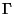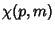## Hilbert Polynomial

Letbe an Algebraic Curve in a projective space of Dimension, and letbe the Prime Ideal defining, and letbe the number of linearly independent forms of degreemodulo. For large,is a Polynomial known as the Hilbert polynomial.

References

Iyanaga, S. and Kawada, Y. (Eds.). Encyclopedic Dictionary of Mathematics. Cambridge, MA: MIT Press, p. 36, 1980.